# Plotting bar charts in Jupyter¶

Craig Stuart Sapp
3 May 2018

This jupyter notebook demonstrates how to load data from Humdrum files extracted with a command pipeline for plotting or otherwise analyzing the data further within the notebook. Plotting is done using the pandas library.

Note that humdrum tools has to be installed already and visible in the command-path to run the above command pipeline.

Below is an example of how to load data into jupyter from a shell command. The command follows the ! character near the start of the line in the cell below. The result of the pipeline is stored as a list of strings, one for each line of text, in a variable called input. The second line of the following cell causes the first 20 entries of the input variable to be shown, which is useful for checking on problems within the command pipeline.

In :
input = ! humcat -s h://chorales | deg -at | serialize | ridx -H | egrep -v "=|r"
print(input[0:20])

['1', '1', '6', '7', '1', '5', '6', '4', '3', '2', '1', '5', '1', '7', '1', '2', '3', '4', '5', '1']


The steps along the command pipeline are:

command

meaning

[humcat](http://extras.humdrum.org/man/humcat) -s h://chorales

Download all of the Bach chorales from the kernscores website as a single stream of Humdrum data segments.

deg -at

Convert the **kern data into scale degrees. The -a option removes direction information from the previous note, and the -t option does not repeat the scale degree information on a secondary tied notes.

serialize

Convert the polyphonic score into a serial sequence of parts.

ridx -H

Remove Humdrum syntax, leaving only data tokens (no null data token lines either).

egrep -v "=|r"

Remove text lines representing barlines or rests.

The data extracted from the Bach chorales is a list of the scale degrees for all notes in the h://chorales data set. In other words, given the primary key of each chorale (not keeping track of modulations), the extracted data contains information about counts of notes by scale degree.

To see a list of all unique tokens in the input list:

In :
unique = sorted(list(set(input)))
print(unique)

['1', '1+', '1-', '2', '2+', '2-', '3', '3+', '3-', '4', '4+', '4-', '5', '5+', '5-', '6', '6+', '6-', '7', '7-']


Scale degrees with a minus sign after them mean a semitone lower than in the scale (such as 3- meaning E-flat in C major, where 3 means E). And a plus following the scale-degree digit means higher by a semitone. Notice that one scale degree alteration case is missing...

## Plotting frequency data with pandas¶

The pandas library is useful for working with arrays of data, and it also has an interface to matplotlib, which is the main plotting library for python. To use pandas, first load the library with the following command:

In :
import pandas as pd


Now that the data has been loaded into the notebook in the previous section, convert the input list into a pandas Series object, and then plot the note counts for each scale degree:

In :
series = pd.Series(data=input)

In :
ax = series.value_counts().plot.bar(title="Scale degrees used in Bach chorales")
ax.set_xlabel("scale degree")
ax.set_ylabel("number of note attacks");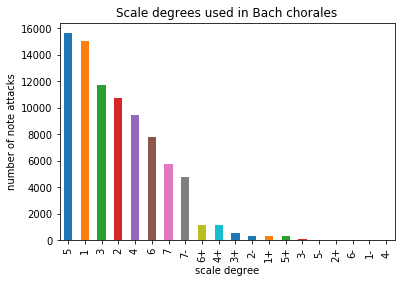## Changing the plot size¶

Here is an example of how to change the size of the plot by adding the figsize parameter to bar(). The units of the width and height values are in inches (10 inches wide and 3 inches high), although the plot will be scaled to the width of the cell if it is too large to fit. Also notice a variant method of how a title can be added to the plot.

In :
ax = series.value_counts().plot.bar(figsize=(10, 3))
ax.set_title("Scale degrees used in Bach chorales")
ax.set_xlabel("scale degree")
ax.set_ylabel("number of note attacks");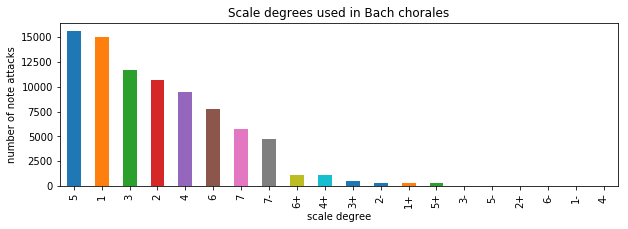## Fractional counts on the y-axis¶

Sometimes it is useful to normalize the counts of each category, such that the sum of all y-values is 1. This allows for numeric comparisons between plots that have different overall count totals. To display the counts as fractional frequencies, add normalize=True to the value_counts() function. This example also demonstrates how to set the bars to a constant color.

In :
ax = (series.value_counts(normalize=True)
.plot.bar(rot=0, figsize=(10,4), color="#ccddee"))
ax.set_xlabel("scale degree")
ax.set_ylabel("number of note attacks (fraction)")
ax.set_title("Scale degrees used in Bach chorales");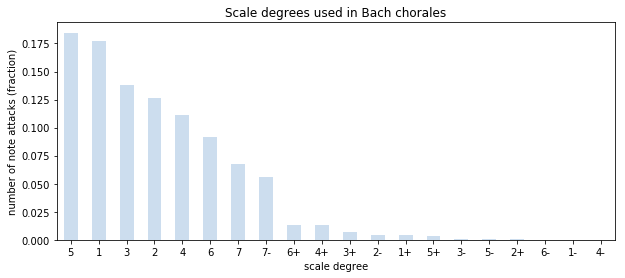## Logarithmic scaling of axes¶

The rare scale-degree cases are hard to see, so if needed, you can scale the vertical axis logarithmically like this:

In :
ax = series.value_counts().plot.bar(logy=True)
ax.set_xlabel("scale degree")
ax.set_ylabel("number of note attacks")
ax.set_title("Scale degrees used in Bach chorales");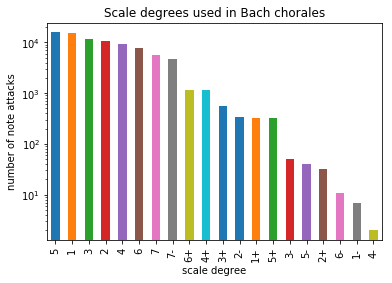## Horizontal orientation of bars¶

Another plot variant is to display the categories horizontally using the barh plotting function:

In :
ax = series.value_counts(ascending=True).plot.barh(logx=True);
ax.set_ylabel("scale degree")
ax.set_xlabel("number of note attacks (log scale)")
ax.set_title("Scale degrees used in Bach chorales");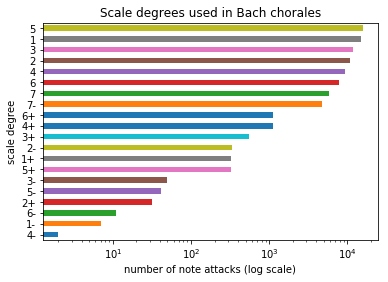## Sorting categories by scale degree¶

This section describes how to sort categories on the plot by scale degree rather than frequency of the scale degrees. First, define a function, called sdorder, that sorts the scale degrees in a musical fashion:

In :
import re

def sdorder(s):
p = re.compile("([0-9]+)([+-]?)")
m = p.match(s)
v = int(m.group(1)) * 10;
if m.group(2) == '+':
v += 1
elif m.group(2) == '-':
v -= 1
return v

sdsequence = sorted(series.unique(), key=sdorder)
print(sdsequence)

['1-', '1', '1+', '2-', '2', '2+', '3-', '3', '3+', '4-', '4', '4+', '5-', '5', '5+', '6-', '6', '6+', '7-', '7']


Then with the list of unique sorted scale-degree values, sdsequence, use one of the following two forms to plot the data in scale degree order:

In :
sdcategories = pd.api.types.CategoricalDtype(categories=sdsequence, ordered=True)
newseries = series.astype(sdcategories)
newseries.value_counts().sort_index().plot.bar();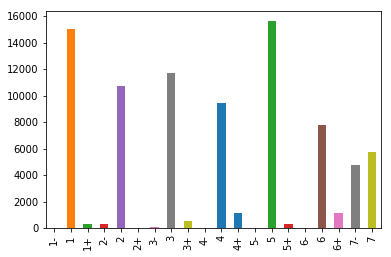In :
newseries = pd.Series(pd.Categorical(input, ordered=True, categories=sdsequence))
newseries.value_counts().sort_index().plot.bar();## Saving figures to files¶

Plots can be saved to a file located in the same directory as the working/starting directory for the notebook (if the notebook is located on the desktop, the saved figures will be placed on the desktop). Notice how the output format is controlled by the filename extension.

In :
newseries = pd.Series(pd.Categorical(input, ordered=True, categories=sdsequence))
ax = newseries.value_counts().sort_index().plot.bar();
ax.get_figure().savefig("myplot.png")
ax.get_figure().savefig("myplot.svg")
ax.get_figure().savefig("myplot.pdf")## Plotting scale degrees by vocal part¶

First extract the scale degree data by voice from the chorales. Data for individual voices are extracted using the extractx Humdrum Extras command. All of the chorales are organized so that the first spine is the bass part, the tenor is the second, the alto is the third, and the soprano is the fourth. Alternatively, the bass part could be extracted with the command extractx -g Ibass which would extract the part(s) that contain the string Ibass (from the instrument label interpretation). Likewise extractx -g I\"Bass can also be used to extract the part based on the instrumental name.

In :
input_bass = ! humcat -s h://chorales| extractx -s 1 | deg -at | serialize | ridx -H | egrep -v "=|r"
input_tenor = ! humcat -s h://chorales| extractx -s 2 | deg -at | serialize | ridx -H | egrep -v "=|r"
input_alto = ! humcat -s h://chorales| extractx -s 3 | deg -at | serialize | ridx -H | egrep -v "=|r"
input_soprano = ! humcat -s h://chorales| extractx -s 4 | deg -at | serialize | ridx -H | egrep -v "=|r"


Then create four panadas series for the data sorted by scale degree:

In :
voices = [None] * 4
voices = pd.Series(pd.Categorical(input_soprano, ordered=True, categories=sdsequence))
voices = pd.Series(pd.Categorical(input_alto, ordered=True, categories=sdsequence))
voices = pd.Series(pd.Categorical(input_tenor, ordered=True, categories=sdsequence))
voices = pd.Series(pd.Categorical(input_bass, ordered=True, categories=sdsequence))


Finally create a figure with four subplots and fill it in:

In :
import matplotlib.pyplot as plt
fig, axes = plt.subplots(nrows=2, ncols=2)
ax0 = voices.value_counts().sort_index().plot.bar(ax=axes[0,0])
ax1 = voices.value_counts().sort_index().plot.bar(ax=axes[0,1])
ax2 = voices.value_counts().sort_index().plot.bar(ax=axes[1,0])
ax3 = voices.value_counts().sort_index().plot.bar(ax=axes[1,1])
ax0.set_title("soprano")
ax1.set_title("alto")
ax2.set_title("tenor")
ax3.set_title("bass")
fig.suptitle("Scale-degree counts in Bach chorales by voice")
fig.tight_layout(rect=[0,0.03,1,0.95])
fig.set_size_inches(10,5)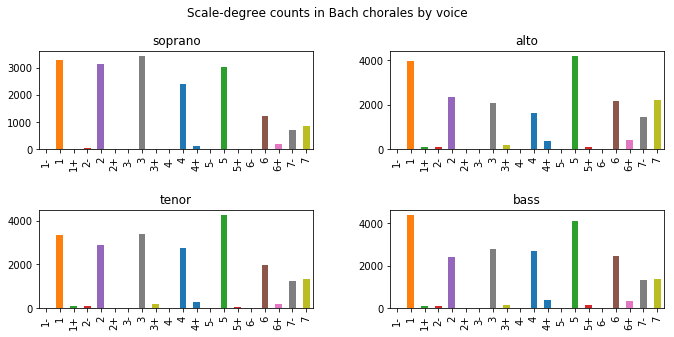Notice that the soprano part focuses on scale degrees 2, 3, and 4 much more than the alto or bass parts do. The soprano focuses on the third slightly more than the root, and the tenor noticeably focuses on the 5th scale degree more than other voices.

The previous examples always download the entire Bach chorale dataset every time they are needed for one of the command pipelines. This does not happen too often (only 5 times in all of the above cells), but if you need to store a local copy, this section demonstrates how to do that. In the following cell a directory called ~/Desktop/mychorales is created, then the working location is changed to that directory, and then the chorales are downloaded with the humsplit command. The first two commands are magic commands for jupyter, but could also be done as regular shell commands like the third command, prefixing the command with ! rather than %.

In :
%mkdir -p ~/Desktop/mychorales
%cd ~/Desktop/mychorales
!humsplit h://chorales
filelist = ! ls *.krn
print(filelist[0:5])
print("Number of files:", len(filelist))

['chor001.krn', 'chor002.krn', 'chor003.krn', 'chor004.krn', 'chor005.krn']
Number of files: 370


Now the command pipeline can access the local copy of the chorales for more intensive, and probably faster, use:

In :
localinput = ! cat *.krn | deg -at | serialize | ridx -H | egrep -v "=|r"
print(localinput[0:20])

['1', '1', '6', '7', '1', '5', '6', '4', '3', '2', '1', '5', '1', '7', '1', '2', '3', '4', '5', '1']


Alternatively, you can access the files by indicating the directory that they are stored in, rather than depending on being within a particular directory:

In :
localinput2 = ! humcat -s ~/Desktop/mychorales/*.krn | deg -at | serialize | ridx -H | egrep -v "=|r"
print(localinput2[0:20])

['1', '1', '6', '7', '1', '5', '6', '4', '3', '2', '1', '5', '1', '7', '1', '2', '3', '4', '5', '1']


## Comparison of major/minor modes¶

This section demonstrates how to group works by mode into major and minor key groups. In the previous sections, pitches were processed by scale degrees, but in this section they are processed by pitch classes in terms of semitones relative to the tonic (first) note of the key. For example the tonic is assigned to be 0, the second scale degree is 2, since it is two semitones above the tonic pitch; the 5th scale degree is assigned 7, and so on.

First, create separate lists for the major and minor chorale filenames. There are 177 chorales in the minor mode, and 193 in the major mode:

In :
minorfiles = ! egrep -l '^\*[a-g][-#]*:' ~/Desktop/mychorales/*.krn
len(minorfiles)

Out:
177
In :
majorfiles = ! egrep -l '^\*[A-G][-#]*:' ~/Desktop/mychorales/*.krn
len(majorfiles)

Out:
193
In :
len(minorfiles) + len(majorfiles)

Out:
370

Notice the regular expression '^\*[a-g][-#]*:' which will match to minor key designations in the files. For example C minor is expressed in **kern data as "*c:", and B-flat major is "*B-:". The regular expression will match strings in this form with "^" matching to the start of the line, "\*" matching to the asterisk (needs a backslash since an asterisk by itself has a special meaning), then [a-g] will match to one of the characters in the set (a, b, c, d, e, f, g); the [-#]* means zero or more of the characters - or #, followed by a regular colon character, :.

Now that lists for the major and minor mode files have been created, use those lists to extract the pitch-class information for each subset of the chorales. New commands in the pipeline are:

command

meaning

[cat](https://www.tecmint.com/13-basic-cat-command-examples-in-linux) \$filelist Concatenate all of the filenames in the$filelist python string variable.

[transpose](http://extras.humdrum.org/man/transpose) -k c

Transpose the music to the key of C major/minor (requires a key designation in the files).

[kern](http://www.humdrum.org/Humdrum/commands/kern.html) -x

Remove all non-pitch information from **kern spine data.

[pc](http://www.humdrum.org/Humdrum/commands/pc.html) -x

Convert pitches into pitch-class numbers (C=0, C-sharp/D-flat=1, D=2, etc.)

[egrep](https://www.computerhope.com/unix/uegrep.htm) -v "[]=r_]"

Remove barlines (=), rests (r), ending tied notes (]) and intermediate tied notes(_).

[sed](http://www.grymoire.com/Unix/Sed.html) 's/\[//g'

Remove tie-start marker from analysis data.

In :
filelist = " ".join(majorfiles)
majorinput = ! cat $filelist | transpose -k c | kern -x | pc \ | serialize | ridx -H | egrep -v "[]=r_]" | sed 's/\[//g' print(majorinput[0:10])  ['0', '0', '9', '11', '0', '7', '9', '5', '4', '2']  In : filelist = " ".join(minorfiles) minorinput = ! cat$filelist | transpose -k c | kern -x | pc \
| serialize | ridx -H | egrep -v "[]=|r_]" | sed 's/\[//g'
print(minorinput[0:10])

['7', '0', '2', '3', '2', '0', '11', '0', '7', '2']


For sorting purposes when creating the plots further below, the pitch classes should be converted from strings into integers. This is done with the "dtype=int" option when creating the pandas series:

In :
majorseries = pd.Series(data=majorinput, dtype=int)
minorseries = pd.Series(data=minorinput, dtype=int)


Now plot counts of the pitch-class/scale-degree data for each mode. There are two plots generated for each mode (1) a list of the counts sorted by occurrence, and (2) a list in chromatic order. Notice in particular that the third scale degree occurs at pc=4 in major modes, but pc=3 in minor modes.

In :
import matplotlib.pyplot as plt
fig, axes = plt.subplots(ncols=2)
ax0 = majorseries.value_counts().plot.bar(ax=axes, color="#aa99ee")
ax1 = majorseries.value_counts().sort_index().plot.bar(ax=axes, color="#aa99ee")
ax0.set_title("sorted by occurrence")
ax1.set_title("sorted by pitch class")
ax0.set_xlabel("pitch class semitones relative to tonic note")
ax1.set_xlabel("pitch class semitones relative to tonic note")
ax0.set_ylabel("note counts")
fig.suptitle("PC scale-degree counts in major Bach chorales")
fig.set_size_inches(12,4)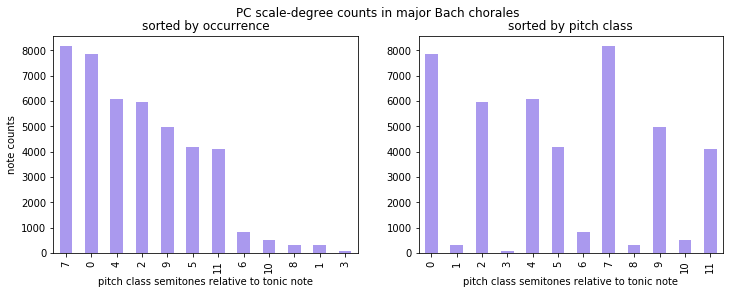In :
fig, axes = plt.subplots(ncols=2)
ax0 = minorseries.value_counts().plot.bar(ax=axes, color="#aa99ee")
ax1 = minorseries.value_counts().sort_index().plot.bar(ax=axes, color="#aa99ee")
ax0.set_title("sorted by occurrence")
ax1.set_title("sorted by pitch class")
ax0.set_xlabel("pitch class semitones relative to tonic note")
ax1.set_xlabel("pitch class semitones relative to tonic note")
ax0.set_ylabel("note counts")
fig.suptitle("PC scale-degree counts in minor Bach chorales")
fig.set_size_inches(12,4)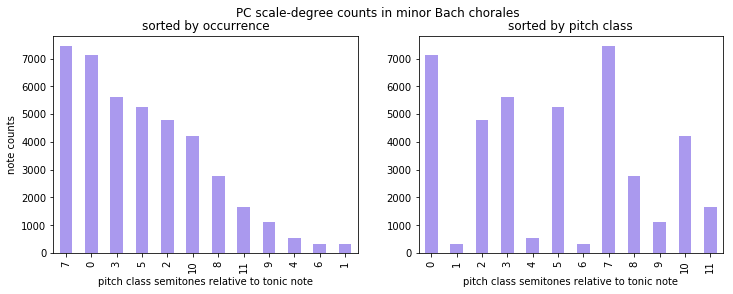Plotting the major/minor plots side by side for each variant:

In :
fig, axes = plt.subplots(ncols=2)
ax0 = majorseries.value_counts().plot.bar(ax=axes, color="#aa99ee")
ax1 = minorseries.value_counts().plot.bar(ax=axes, color="#aa99ee")
ax0.set_title("Major keys")
ax1.set_title("Minor keys")
ax0.set_xlabel("pitch-class semitones relative to tonic note")
ax1.set_xlabel("pitch-class semitones relative to tonic note")
ax0.set_ylabel("note counts")
fig.suptitle("PC scale-degree counts in Bach chorales")
fig.set_size_inches(12,4)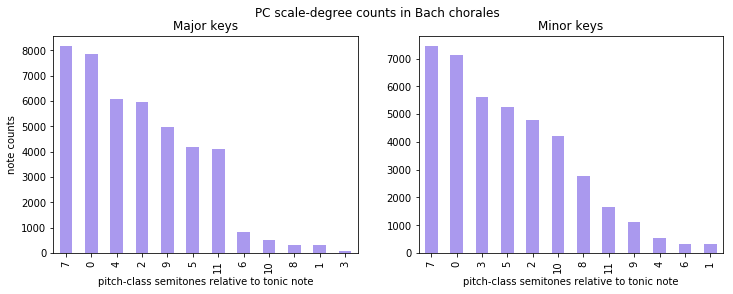In :
fig, axes = plt.subplots(ncols=2)
ax0 = majorseries.value_counts().sort_index().plot.bar(ax=axes, color="#aa99ee")
ax1 = minorseries.value_counts().sort_index().plot.bar(ax=axes, color="#aa99ee")
ax0.set_title("Major keys")
ax1.set_title("Minor keys")
ax0.set_xlabel("pitch-class semitones relative to tonic note")
ax1.set_xlabel("pitch-class semitones relative to tonic note")
ax0.set_ylabel("note counts")
fig.suptitle("PC scale-degree counts in Bach chorales")
fig.set_size_inches(12,4)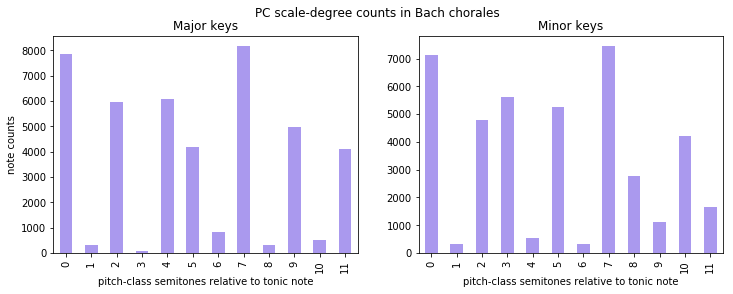Below is an example of stacked plots to merge the two above plots into a single plot. First the major and minor counts are extracted to separate lists:

In :
majorhist = majorseries.value_counts().sort_index().tolist()
majorhist

Out:
[7876, 316, 5946, 88, 6078, 4181, 836, 8176, 333, 4977, 521, 4106]
In :
minorhist = minorseries.value_counts().sort_index().tolist()
minorhist

Out:
[7115, 337, 4775, 5632, 555, 5254, 343, 7452, 2784, 1135, 4207, 1643]

Normalize the difference between major and minor notecounts:

In :
df = pd.DataFrame()
df["major"] = majorhist
df["minor"] = minorhist
df["major"] *= 100 / df["major"].sum()
df["minor"] *= 100 / df["minor"].sum()

In :
ax = df.plot.bar(stacked=True, figsize=(10,5))
ax.set_title("Difference between major and minor scale-degree distributions in Bach chorales")
ax.set_xlabel("pitch-class semitones above tonic")
ax.axes.get_yaxis().set_visible(False);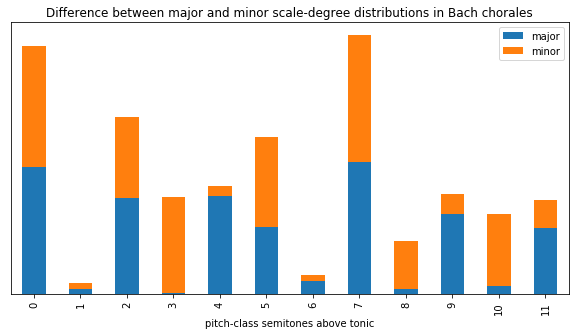The largest difference is that major keys use the 4th semitone above tonic (major 3rd), while the minor keys use the 3rd semitone above the tonic (minor 3rd). Also semitones 8 and 10 are mostly used by minor keys, while 9 and 10 are used by major keys. For major keys, there is more 6, probably functioning as a leading tone to a tonicization of the dominant (7). Minor key chorales also use a little more of the subdominant scale-degree (5).

## Beyond major and minor¶

The Bach choral sets has music in other modes besides major and minor. For the above analyses, modes such as dorian are assigned to the minor category, and mixolydian chorales are assigned to the major category. However, the Bach chorale data set contains mode indications for non-major/-minor modes, such as dorian, phrygian and mixolydian. For example the indication for D dorian is "*d:dor and C mixolydian is "*C:mix. The letter of the pitch name depends on the third scale degree of the mode: a minor third will use a lower-cases tonic letter, since it is more similar to minor keys and to major ones. But in the following calls to egrep the -i option is used to ignore the letter cases.

Here are the counts of chorales in non-major/-minor modes:

In :
# dorian mode
dorfiles = ! egrep -il '^\*[a-g][-#]*:dor' ~/Desktop/mychorales/*.krn
len(dorfiles)

Out:
37
In :
# phrygian mode
phrfiles = ! egrep -il '^\*[a-g][-#]*:phr' ~/Desktop/mychorales/*.krn
len(phrfiles)

Out:
2
In :
# lydian mode
lydfiles = ! egrep -il '^\*[a-g][-#]*:lyd' ~/Desktop/mychorales/*.krn
len(lydfiles)

Out:
0
In :
# mixolydian mode
mixfiles = ! egrep -il '^\*[a-g][-#]*:mix' ~/Desktop/mychorales/*.krn
len(mixfiles)

Out:
9
In :
# locrian mode
locfiles = ! egrep -il '^\*[a-g][-#]*:loc' ~/Desktop/mychorales/*.krn
len(locfiles)

Out:
0
In :
# all non-major/-minor keys
modefiles = ! egrep -il '^\*[a-g][-#]*:[a-z][a-z][a-z]' ~/Desktop/mychorales/*.krn
len(modefiles)

Out:
48

Add the data from the 37 dorian files to the stacked scale-degree plot:

In :
filelist = " ".join(dorfiles)
dorinput = ! cat \$filelist | transpose -k c | kern -x | pc \
| serialize | ridx -H | egrep -v "[]=|r_]" | sed 's/\[//g'
dorseries = pd.Series(data=dorinput, dtype=int)
dorhist = dorseries.value_counts().sort_index().tolist()
df["dorian"] = dorhist
df["dorian"] *= 100 / df["dorian"].sum()
ax = df.plot.bar(stacked=True, figsize=(10,5))
ax.set_title("Relative differences between major, minor and dorian scale degrees in Bach chorales")
ax.set_xlabel("pitch-class semitones above tonic")
ax.axes.get_yaxis().set_visible(False)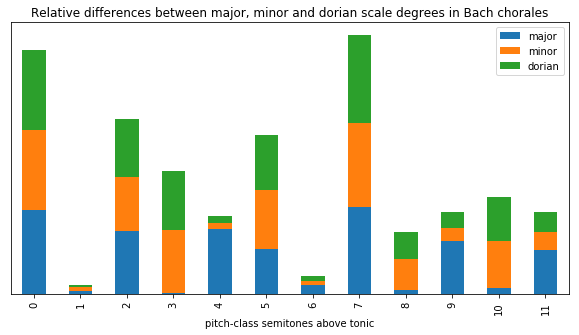Here is a table of the data used to produce the plots. Each column sums to 100%.

In :
df

Out:
major minor dorian
0 18.133260 17.256015 17.264121
1 0.727541 0.817326 0.510584
2 13.689736 11.580811 12.498670
3 0.202606 13.659294 12.785874
4 13.993646 1.346042 1.404106
5 9.626099 12.742530 11.988086
6 1.924759 0.831878 1.233911
7 18.823963 18.073341 18.966067
8 0.766680 6.752037 5.924902
9 11.458765 2.752716 3.467716
10 1.199521 10.203240 9.584087
11 9.453424 3.984769 4.371875

Try using the above data for dorian modes in keycor.